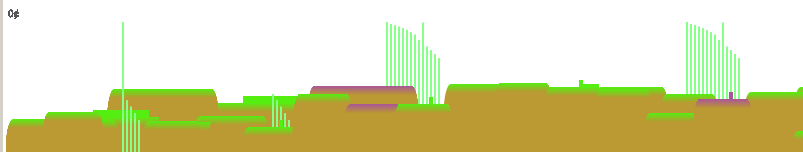# JS1K

Marijn Haverbeke (@marijnjh)

(use arrow keys to move through the slides)

(also, this probably only works on modern browsers)

# JS1K

Write a JS demo in 1024 bytes or less

No external dependencies

Ran earlier this year (up to 10 September)

http://js1k.com

# Platform Game

Malte Ubl (@cramforce) had submitted a game with a data-URL based Mario spriteI started with the intent of one-upping that

Things got out of hand

# The World

Easiest way to model a world is a heightmap# The World

Randomize it a bit# The World

Add gaps# The World

Better gap heuristic# The World

Plant a tree on every third block# The World

This generates the heightmap (52 bytes):

`for(i=0;i<1e4;)  L=H[i++]=i<9|L<w&R()<.3?w:R()*u+80|0;`

`H` = the heightmap
`u` = block width (50)
`w` = screen width (placeholder value for gaps)
`R` = `Math.random`

# Bitmaps

I initially had data-URL bitmaps

Really big for any non-trivial graphics

(This explains the non-existent gameplay in the Mario game)

# Bitmaps

Next attempt: strings of bit-packed pixels

7 bits per char (to ensure we don't get multi-byte UTF-8 sequences)

.... cute, still too big

# Vector Graphics, Then

`Canvas`, obviously

But... `quadraticCurveTo`, `createLinearGradient`...

The method names are just too long

# Mechanized Abbreviation

`for(\$ in C=c.getContext('2d'))  C[\$+(\$||'')]=C[\$];`

We now have `C.qt` for `quadraticCurveTo`, `C.f` for `fill`

Don't try this at home

# Graphics

Solution: everything is a circle

# Graphics

Background is a big circle

# Graphics

Concentric circles make a rainbow...

# Graphics

Clouds are three circles in a row...# Graphics

And the player is... an eye(With iris movement depending on current speed)

# The Game

(click the frame to focus it)

# The Game (in IE9)(But this time it's not IE's fault)

# The Code

```c=document.body.children;h=t=150;L=w=c.width=800;u=D=50;H=[];R=Math.random;for(\$ in C=
c.getContext('2d'))C[\$[J=X=Y=0]+(\$||'')]=C[\$];setInterval("if(D)for(x=405,i=y=I=0;i<1e4;)L=\
H[i++]=i<9|L<w&R()<.3?w:R()*u+80|0;\$=++t%99-u;\$=\$*\$/8+20;y+=Y;x+=y-H[(x+X)/u|0]>9?0:X;j=H[o=\
x/u|0];Y=y<j|Y<0?Y+1:(y=j,J?-10:0);with(C){A=function(c,x,y,r){r&&a(x,y,r,0,7,0);fillStyle=c.P\
?c:'#'+'ceff99ff78f86eeaaffffd45333'.substr(c*3,3);f();ba()};for(D=Z=0;Z<21;Z++){Z<7&&A(Z%6,w/\
2,235,Z?250-15*Z:w);i=o-5+Z;S=x-i*u;B=S>9&S<41;ta(u-S,0);G=cL(0,T=H[i],0,T+9);T%6||(A(2,25,T-7\
,5),y^j||B&&(H[i]-=.1,I++));G.P=G.addColorStop;G.P(0,i%7?'#7e3':(i^o||y^T||(y=H[i]+=\$/99),\
'#c7a'\));G.P(1,'#ca6');i%4&&A(6,t/2%200,9,i%2?27:33);m(-6,h);qt(-6,T,3,T);l(47,T);qt(56,T,56,\
h);A(G);i%3?0:T<w?(A(G,33,T-15,10),fc(31,T-7,4,9)):(A(7,25,\$,9),A(G,25,\$,5),fc(24,\$,2,h),D=B&y\
>\$-9?1:D);ta(S-u,0)}A(6,u,y-9,11);A(5,M=u+X*.7,Q=y-9+Y/5,8);A(8,M,Q,5);fx(I+'¢',5,15)}D=y>h?1:D"
,u);onkeydown=onkeyup=function(e){E=e.type?4:0;e=e.keyCode;J=e^38?J:E;X=e^37?e^39?X:E:-E}```